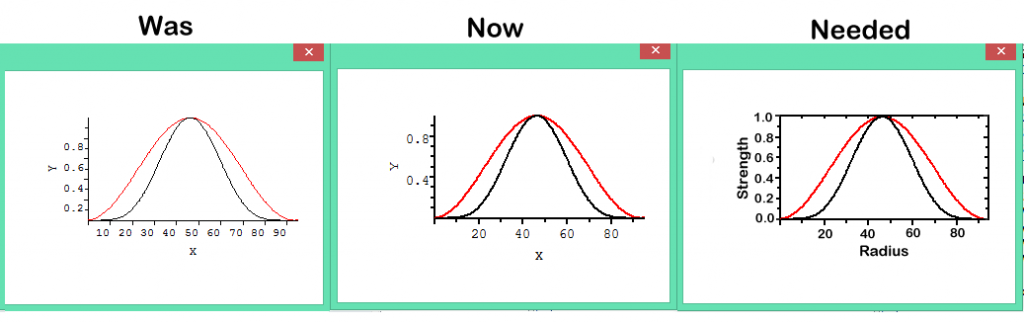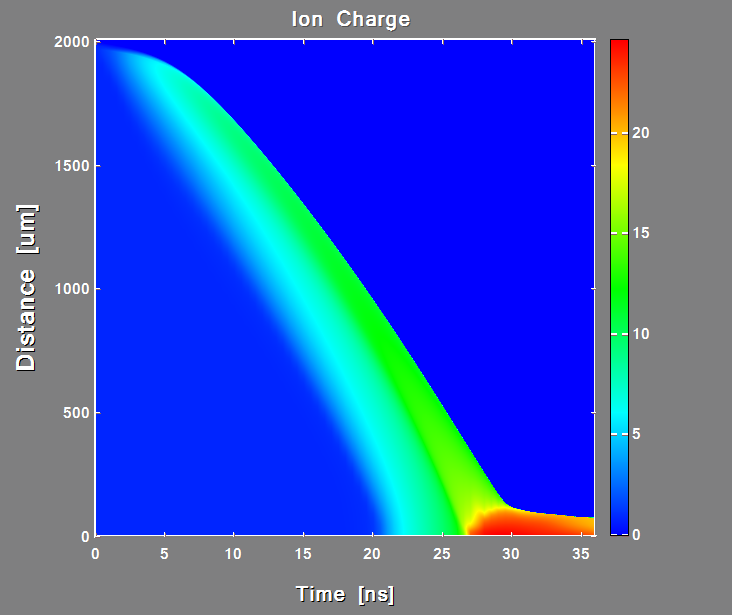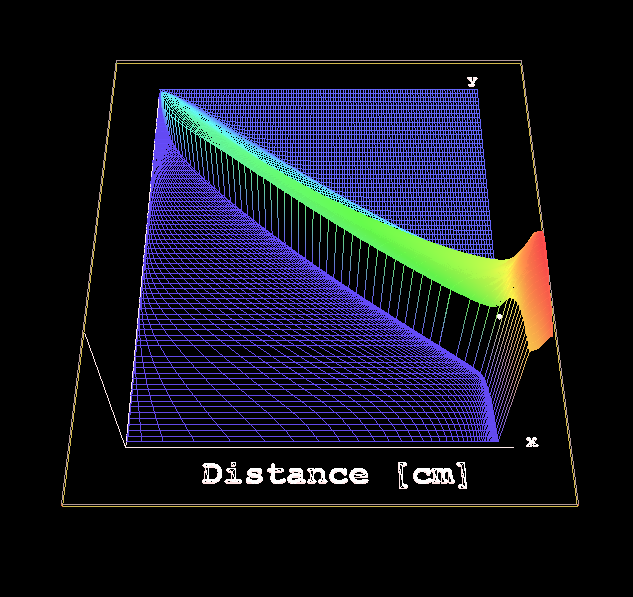Native %pl Goto page Previous  1, 2, 3, 4, 5, 6, 7, 8, 9  Next
Author Message
aebolzan

Joined: 06 Jul 2007
Posts: 229
Location: La Plata, ArgentinaPosted: Thu Nov 17, 2016 11:43 am    Post subject:Sorry!...by mistake I did not copy those files to the "include" folder but to the FTN95 one.......that's why the compiler did not find the subroutine...now all works fine, thanks!....I will try now with my own code.. AgustinDanRRightJoined: 10 Mar 2008
Posts: 2209
Location: South Pole, AntarcticaPosted: Thu Nov 17, 2016 7:13 pm    Post subject:Paul, Can you please also create the boxed plotting surface like here ?After finishing with XY plots obvious next logical step will be if new Plotter will also create such surface plots with just user providing data and clicking "run" it will be totally genial utility everyone wantSame for 3D plots (surfaceplot and 3D plots are sharing 99.999% of the source code)PaulLaidlerJoined: 21 Feb 2005
Posts: 6466
Location: Salford, UKPosted: Fri Nov 18, 2016 8:13 am    Post subject:Thanks Dan The box should be easy. The rest maybe next week.aebolzan

Joined: 06 Jul 2007
Posts: 229
Location: La Plata, ArgentinaPosted: Tue Nov 22, 2016 7:19 pm    Post subject:I was trying to run your example program but using the option of x_array, with x_array changing from calc1 to calc2 subroutines.., so I have two data sets, x-y and x-z, so the program code is:

 Code: PROGRAM main       USE mydata       n_points(1) = 0       n_points(2) = 0       i=winio@('%ww[no_border]%ca[Damped wave]%pv&')       i=winio@('%mn[Edit[Draw first,Draw second,Clear]]&', draw1, draw2,clear)       i=winio@('%fn[Tahoma]&')       i=winio@('%ts&', 1.1d0)       i=winio@('%tc&',rgb@(0,0,80))       i=winio@('%it&')       i=winio@('%`bg&',rgb@(230,255,225))       CALL winop@("%pl[native,independent]")       CALL winop@("%pl[n_graphs=2]")          CALL winop@('%pl[title="Sample plot"]')       CALL winop@("%pl[x_axis=Time(Milliseconds)]")       CALL winop@("%pl[y_axis=Amplitude@(-4.0)]")       CALL winop@("%pl[width=1]")       CALL winop@("%pl[smoothing=4]") ! anti-aliasing       CALL winop@("%pl[colour=red,style=2,pen_style=0,x_array]")          CALL winop@("%pl[colour=blue,style=2,pen_style=4,x_array]")          i=winio@("%pl",800,400,n_points,x,y,z)       END

However, the compiler says: too few arguments to satisfy winio@ format (continuation 7). I found some additional issues during plotting but I like to solved this one first....

Agustinaebolzan

Joined: 06 Jul 2007
Posts: 229
Location: La Plata, ArgentinaPosted: Tue Nov 22, 2016 9:41 pm    Post subject:Found the problem!!!.....although this is not clear in the notes (at least for me), seems that one has to provide as many as x_array/y_array pairs as needed, so that, the last statement in the previous code should be:

 Code: i=winio@("%pl",800,400,n_points,x1,y,x2,z)

The other issue that I discovered is that the function Clear() must include now not only y=0.0 and z=0.0, but also x1=0.0, and x2=0.0 or one will observe that the x-axis remains at x=500 forever....

AgustinPaulLaidlerJoined: 21 Feb 2005
Posts: 6466
Location: Salford, UKPosted: Wed Nov 23, 2016 9:05 am    Post subject:Agustin Yes. Maybe there was an error in my sample code. When using the options [independent,x_array], the x and y arrays are in pairs otherwise there is just one x array.DanRRightJoined: 10 Mar 2008
Posts: 2209
Location: South Pole, AntarcticaPosted: Wed Nov 23, 2016 12:24 pm    Post subject: Re:... wrote: i=winio@('%fn[Tahoma]&') i=winio@('%ts&', 1.1d0) i=winio@('%tc&',rgb@(0,0,80)) i=winio@('%it&') i=winio@('%`bg&',rgb@(230,255,225)) CALL winop@("%pl[native,independent]") CALL winop@("%pl[n_graphs=2]") CALL winop@('%pl[title="Sample plot"]') CALL winop@("%pl[x_axis=Time(Milliseconds)]") CALL winop@("%pl[y_axis=Amplitude@(-4.0)]")

Paul,
Why
CALL winop@...
not
i=winop@... ?PaulLaidlerJoined: 21 Feb 2005
Posts: 6466
Location: Salford, UKPosted: Wed Nov 23, 2016 5:19 pm    Post subject:Dan The is nothing useful to return from winop@.DanRRightJoined: 10 Mar 2008
Posts: 2209
Location: South Pole, AntarcticaPosted: Wed Nov 23, 2016 9:10 pm    Post subject:Not all i=winio@ are useful either but some are. Formally these subroutines with CALL and functions with i=winio@ look and feel like they do the same things. Why lose the possibility to have potential respond from function? This i=winio@ could be useful for something in future like generally useful is i=winio@ Besides CALLs break the style and confuse On early stage of development it is possible to change things. Later this will be almost impossible.Last edited by DanRRight on Wed Nov 23, 2016 9:22 pm; edited 1 time in totalPaulLaidlerJoined: 21 Feb 2005
Posts: 6466
Location: Salford, UKPosted: Wed Nov 23, 2016 9:18 pm    Post subject:OK. I will look into that.aebolzan

Joined: 06 Jul 2007
Posts: 229
Location: La Plata, ArgentinaPosted: Thu Nov 24, 2016 5:26 pm    Post subject:Dear Paul, I have found some problems with the locations of tick labels. As I am trying to implement the new %pl for plotting different types of data sets during the run of the program (they can be Current-Time, Potential-Time, Current-Potential, etc.), I have implemented a Function Legend that changes the Axis labels depending on what is plotted at a certain time. The result is that the tick labels can appear correctly located below the x-axis, for instance, but once I plotted a second data set, they move upwards (the same for the y-axis) and remain there for the rest of the run. See this code, for instance: [code]WINAPP MODULE mydata USE clrwin INTEGER n_points(2) REAL*8 xstart(2),dx(2) INTEGER,PARAMETER::n=1000 REAL*8:: y(n)=0.0,z(n)=0.0,w(n) integer*4 :: ancho,alto logical*4 :: plot=.false. CONTAINS INTEGER FUNCTION legend() integer ancho2,alto2,alto3,ancho3 ancho2=ancho/2.0 alto2=alto-20 ancho3=ancho*0.05 alto3=alto/2.0 if(plot) then CALL draw_characters@("Time/s", ancho2, alto2, 0) CALL rotate_font@(90.0D0) CALL draw_characters@("Current/A", ancho3, alto3, 0) CALL rotate_font@(0.0D0) endif legend = 2 END FUNCTION legend SUBROUTINE calc1(k) INTEGER i,x,k DOUBLE PRECISION p1,p2,p3 p1=1.5d0 p2=150.0d0 p3=15d0 x=0 plot=.true. DO i=1,k y(i)=p1*exp(-x/p2) x=x+1 ENDDO END SUBROUTINE calc1 SUBROUTINE calc2(k) INTEGER i,x,k DOUBLE PRECISION p1,p2,p3 p1=1.0d0 p2=150.0d0 p3=20d0 x=0 plot=.true. DO i=1,k z(i)=p1*exp(-x/p2) x=x+1 ENDDO END SUBROUTINE calc2 SUBROUTINE calc3(k) INTEGER i,x,k DOUBLE PRECISION p1,p2,p3 p1=2.0d0 p2=150.0d0 p3=20d0 x=0 plot=.true. DO i=1,k w(i)=p1*exp(-x/p2) x=x+1 ENDDO END SUBROUTINE calc3 INTEGER FUNCTION draw1() CALL calc1(n_points(1)) CALL simpleplot_redraw@() draw1 = 2 END FUNCTION draw1 INTEGER FUNCTION draw2() CALL calc2(n_points(2)) CALL simpleplot_redraw@() draw2 = 2 END FUNCTION draw2 INTEGER FUNCTION clear() y = 0.0d0 z = 0.0d0 w = 0.0d0 plot=.false. CALL simpleplot_redraw@() clear = 2 END FUNCTION clear INTEGER FUNCTION change() INTEGER i if(n_points(2) == 500)then n_points(2) = 1000 dx(2) = 1.0d0 CALL calc3(1000) i = CHANGE_GRAPH_STYLE@(2,0,0,255) i = CHANGE_GRAPH_DATA@(2,0.0d0,w) else n_points(2) = 500 dx(2) = 2.0d0 CALL calc2(500) i = CHANGE_GRAPH_STYLE@(2,2,4,RGB@(0,0,255)) i = CHANGE_GRAPH_DATA@(2,0.0d0,z) endif CALL simpleplot_redraw@() change = 2 END FUNCTION change END MODULE mydata PROGRAM main USE mydata external legend ancho=0.8*clearwin_info@('SCREEN_WIDTH') alto=0.8*clearwin_info@('SCREEN_DEPTH') n_points(1) = 1000 n_points(2) = 500 xstart = 0.0d0 dx(1) = 1.0d0 dx(2) = 2.0d0 i=winio@('%ww[no_border]%ca[Damped wave]%pv&') i=winio@('%mn[Edit[Draw first,Draw second,Change second,ClearPaulLaidlerJoined: 21 Feb 2005
Posts: 6466
Location: Salford, UKPosted: Thu Nov 24, 2016 6:04 pm    Post subject:Thanks. I will take a look at it.aebolzan

Joined: 06 Jul 2007
Posts: 229
Location: La Plata, ArgentinaPosted: Thu Nov 24, 2016 6:36 pm    Post subject:Ooopppss....seems that the code is incomplete. This is the main program:

 Code: PROGRAM main       USE mydata       external legend       ancho=0.8*clearwin_info@('SCREEN_WIDTH')      alto=0.8*clearwin_info@('SCREEN_DEPTH')       n_points(1) = 1000       n_points(2) = 500       xstart = 0.0d0       dx(1) = 1.0d0       dx(2) = 2.0d0       i=winio@('%ww[no_border]%ca[Damped wave]%pv&')       i=winio@('%mn[Edit[Draw first,Draw second,Change second,Clear]]&', draw1, draw2,change,clear)       i=winio@('%fn[Tahoma]&')       i=winio@('%ts&', 1.1d0)       i=winio@('%tc&',rgb@(0,0,80))       i=winio@('%it&')       i=winio@('%`bg&',rgb@(230,255,225))       CALL winop@("%pl[native,independent]")       CALL winop@("%pl[n_graphs=2]")          CALL winop@('%pl[title="Sample plot"]')       CALL winop@("%pl[x_axis=@]")       CALL winop@("%pl[y_axis=@]")       CALL winop@("%pl[width=1]")       CALL winop@("%pl[smoothing=4]") ! anti-aliasing       CALL winop@("%pl[colour=red,style=2,pen_style=0]")          CALL winop@("%pl[colour=blue,style=2,pen_style=4]")          i=winio@("%^pl",ancho,alto,n_points,xstart,dx,y,z,legend)       ENDDanRRightJoined: 10 Mar 2008
Posts: 2209
Location: South Pole, AntarcticaPosted: Thu Nov 24, 2016 8:22 pm    Post subject:What I also missed in %pl and had no explanation why it was not included was keyword Y_MAX=Value. It has Y_MIN=Value but not Y_MAX.Last edited by DanRRight on Thu Nov 24, 2016 8:46 pm; edited 1 time in totalaebolzan

Joined: 06 Jul 2007
Posts: 229
Location: La Plata, ArgentinaPosted: Thu Nov 24, 2016 8:36 pm    Post subject:what I have not checked is if [x_array] and [xmin,dx] are exclusive options.....although it seems so.....more interesting is the fact that the location of ticks follows a very funny code: it is impossible to know what the plot will show....until it appears... Using [x_array] you can select somehow the xmin and xmax limits of the data in a plot, but I also found some strange consequences of this (in the tick labels) that I am still investigating.... AgustinDisplay posts from previous: All Posts1 Day7 Days2 Weeks1 Month3 Months6 Months1 Year Oldest FirstNewest First
 All times are GMT + 1 HourGoto page Previous  1, 2, 3, 4, 5, 6, 7, 8, 9  Next Page 3 of 9